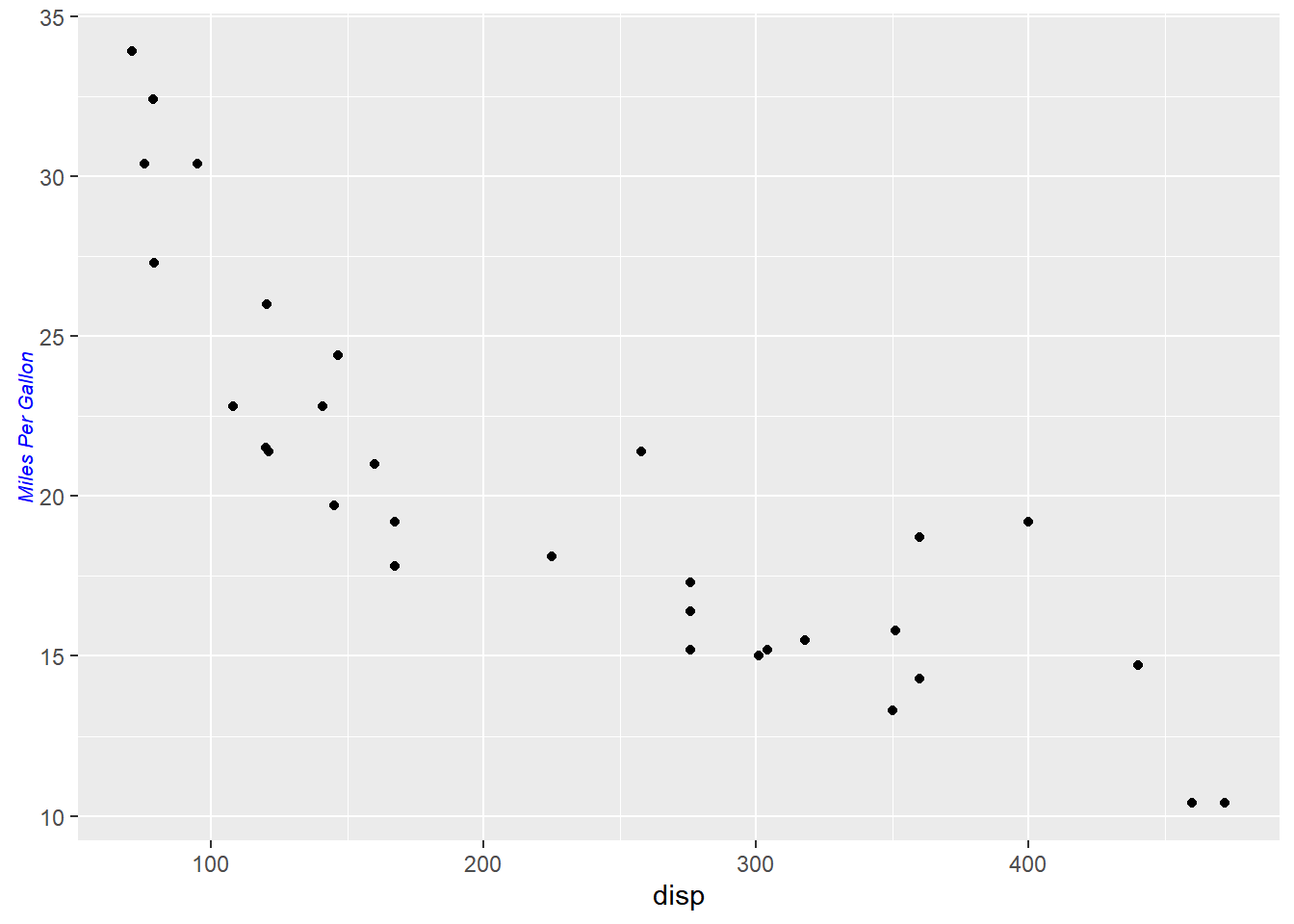# Chapter 4 Labels

## 4.1 Introduction

In this chapter, we will learn to:

• add title and subtitle to the plot
• modify axis labels
• modify axis range
• remove axis
• format axis

## 4.2 Basic Plot

Let us start with a simple scatter plot. We will continue to use the mtcars data set and examine the relationship between displacement and miles per gallon using `geom_point()`.

``````ggplot(mtcars) +
geom_point(aes(disp, mpg))``````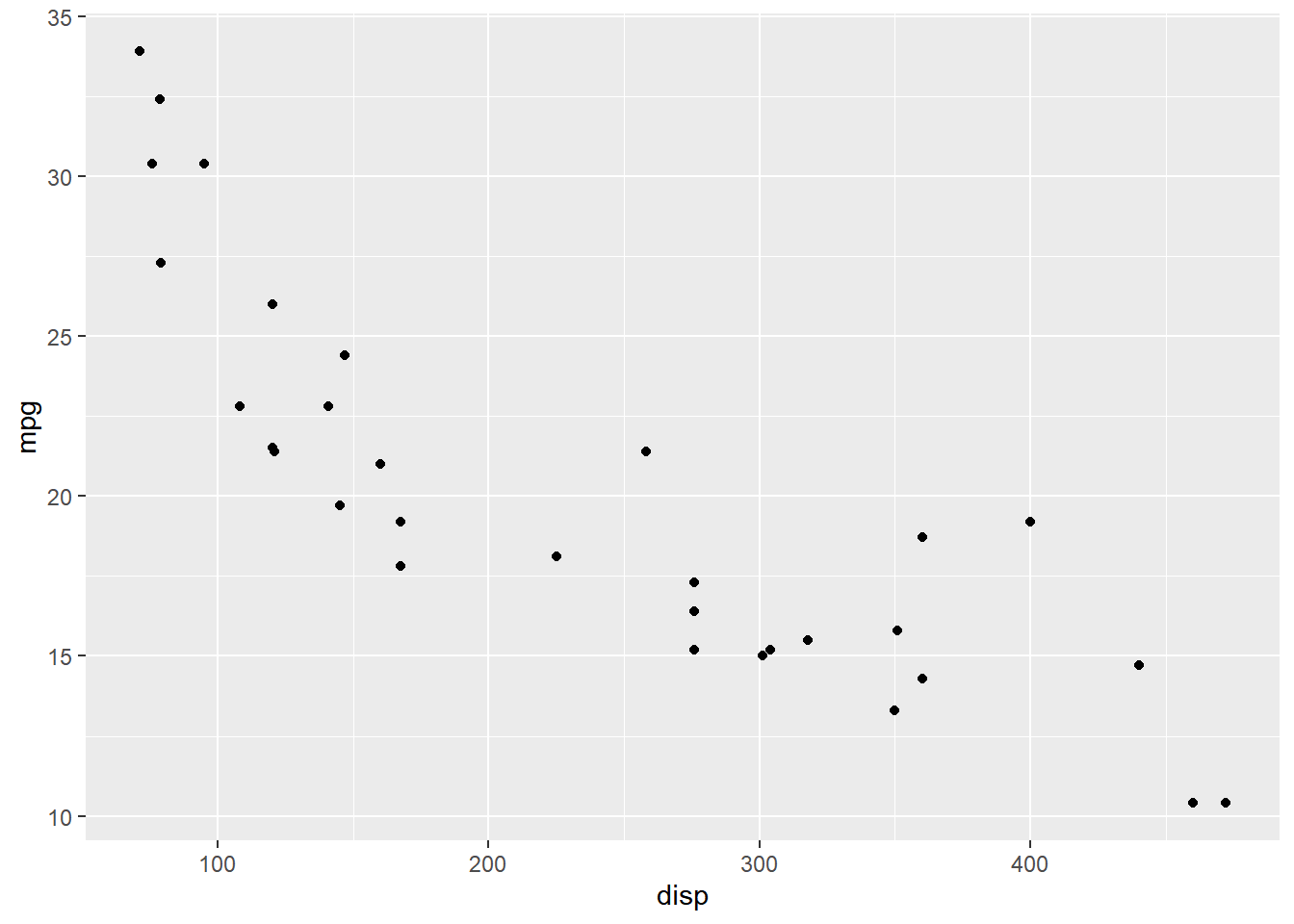## 4.3 Title & Subtitle

There are two ways to add title to a plot:

• `ggtitle()`
• `labs()`

### 4.3.1 ggtitle()

Let us explore the `ggtitle()` function first. It takes two arguments:

• label: title of the plot
• subtitle: subtitle of the plot
``````ggplot(mtcars) +
geom_point(aes(disp, mpg)) +
ggtitle(label = 'Displacement vs Mileage', subtitle = 'disp vs mpg')``````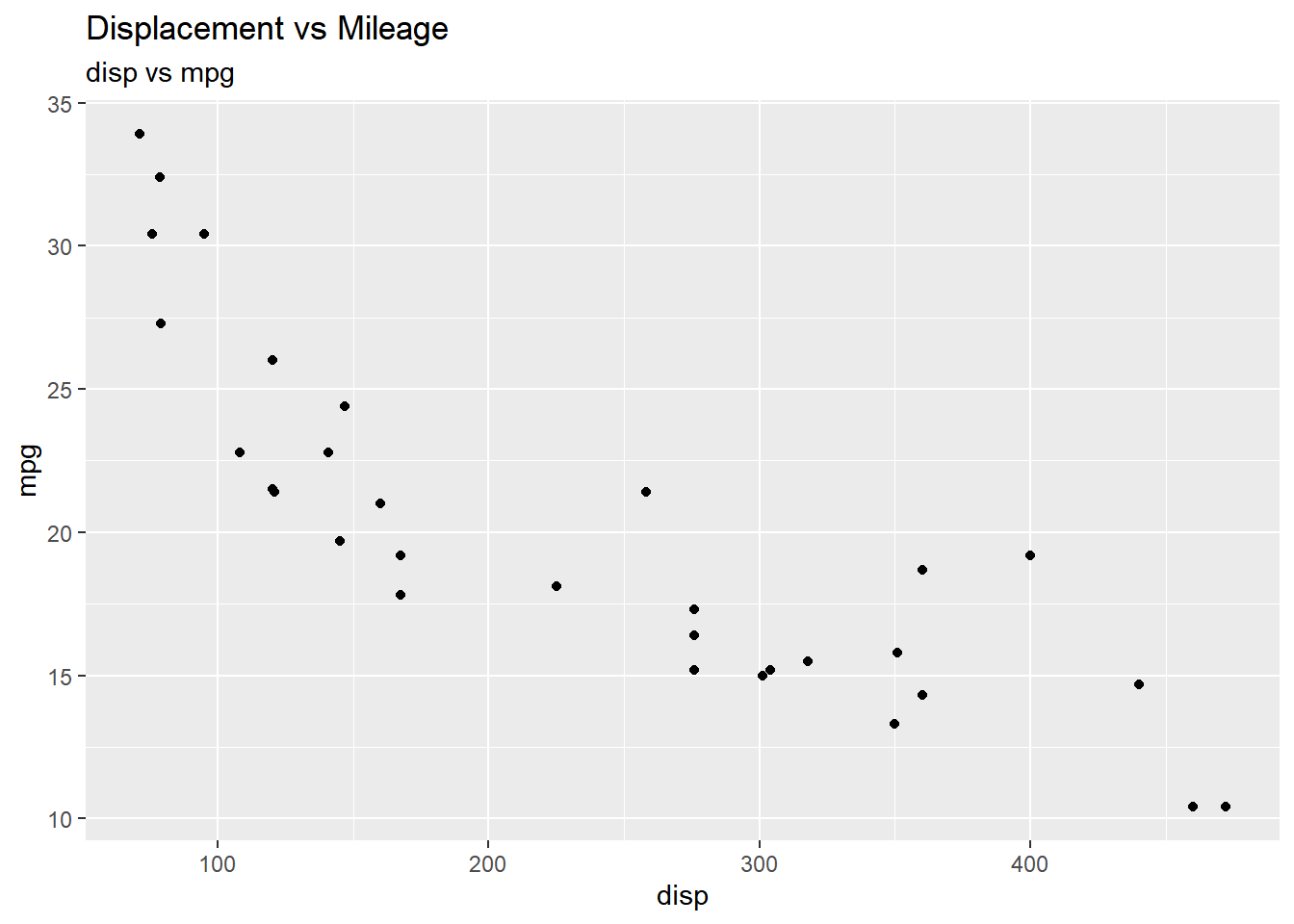## 4.4 Axis Labels

You can add labels to the axis using:

• `xlab()`
• `ylab()`
• `labs()`
``````ggplot(mtcars) +
geom_point(aes(disp, mpg)) +
xlab('Displacement') + ylab('Miles Per Gallon')``````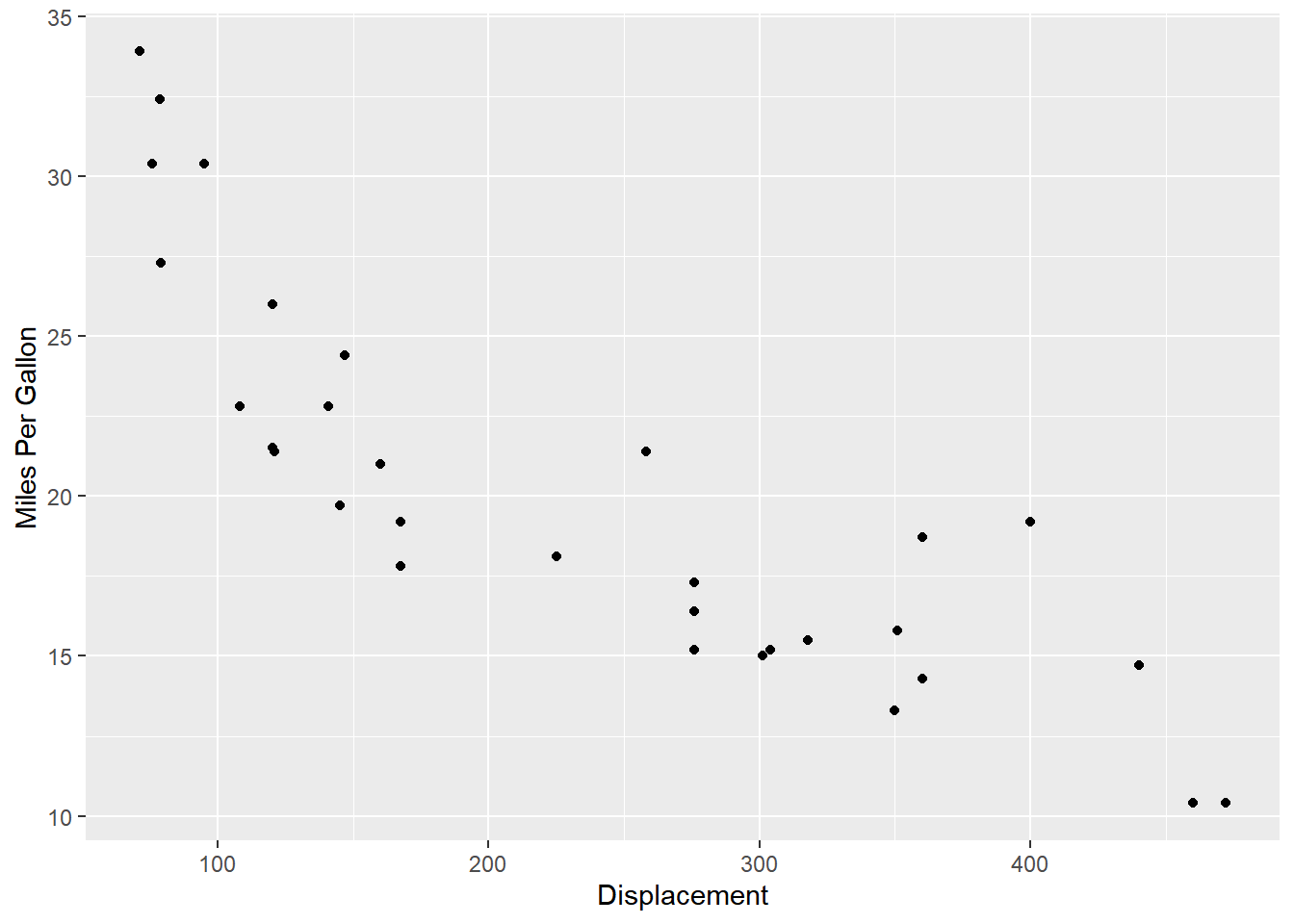## 4.5 Labs

The `labs()` function can be used to add the following to a plot:

• title
• subtitle
• X axis label
• Y axis label
``````ggplot(mtcars) +
geom_point(aes(disp, mpg)) +
labs(title = 'Displacement vs Mileage', subtitle = 'disp vs mpg',
x = 'Displacement', y = 'Miles Per Gallon')``````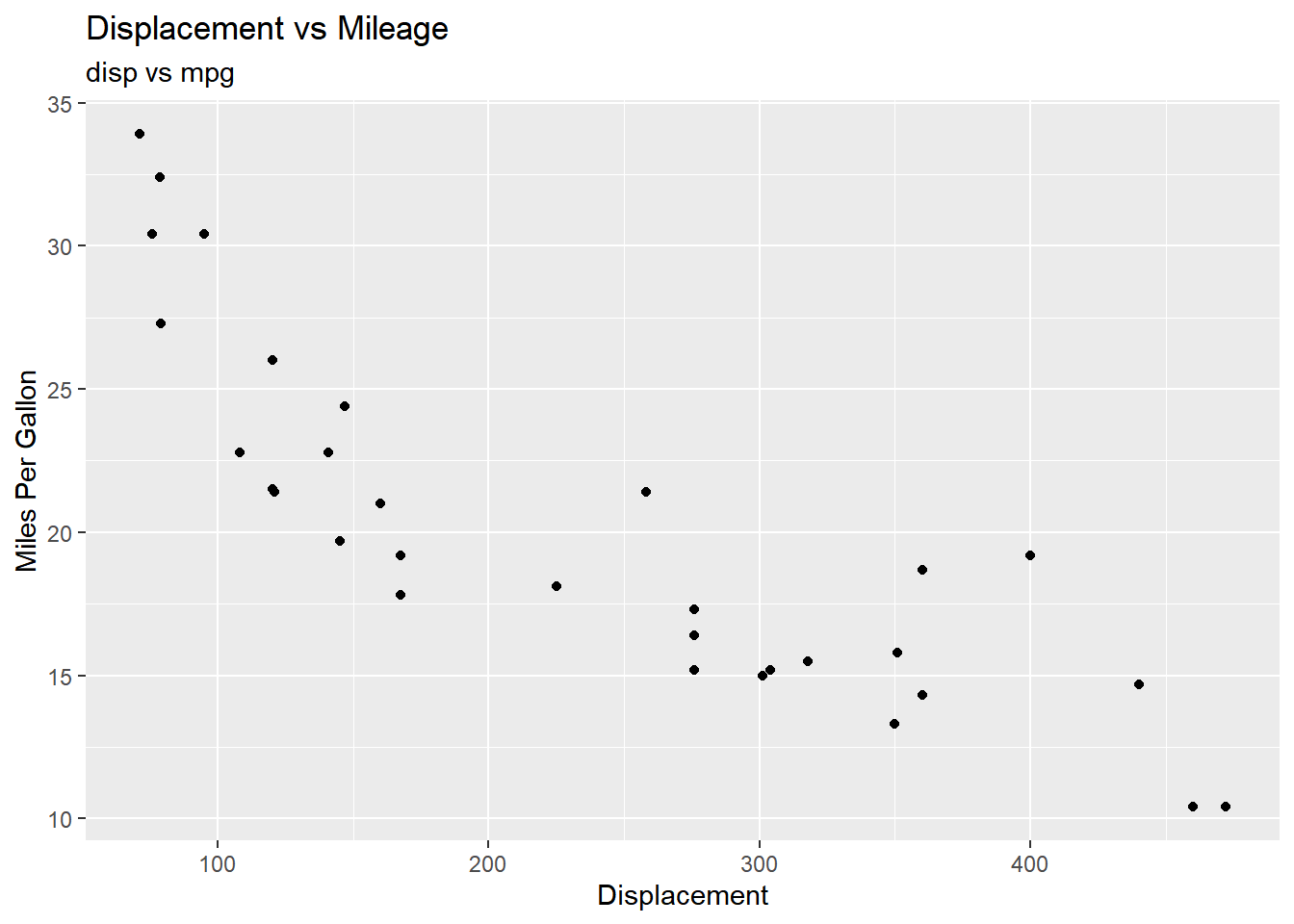## 4.6 Axis Range

In certain scenarios, you may want to modify the range of the axis. In ggplot2, we can achieve this using:

• `xlim()`

• `ylim()`

• `expand_limits()`

• `xlim()` and `ylim()` take a numeric vector of length 2 as input

• `expand_limits()` takes two numeric vectors (each of length 2), one for each axis

• in all of the above functions, the first element represents the lower limit and the second element represents the upper limit

### 4.6.1 X Axis

In the below example, we limit the range of the X axis between `0` and `600` using `xlim`.

``````ggplot(mtcars) +
geom_point(aes(disp, mpg)) +
xlim(c(0, 600))``````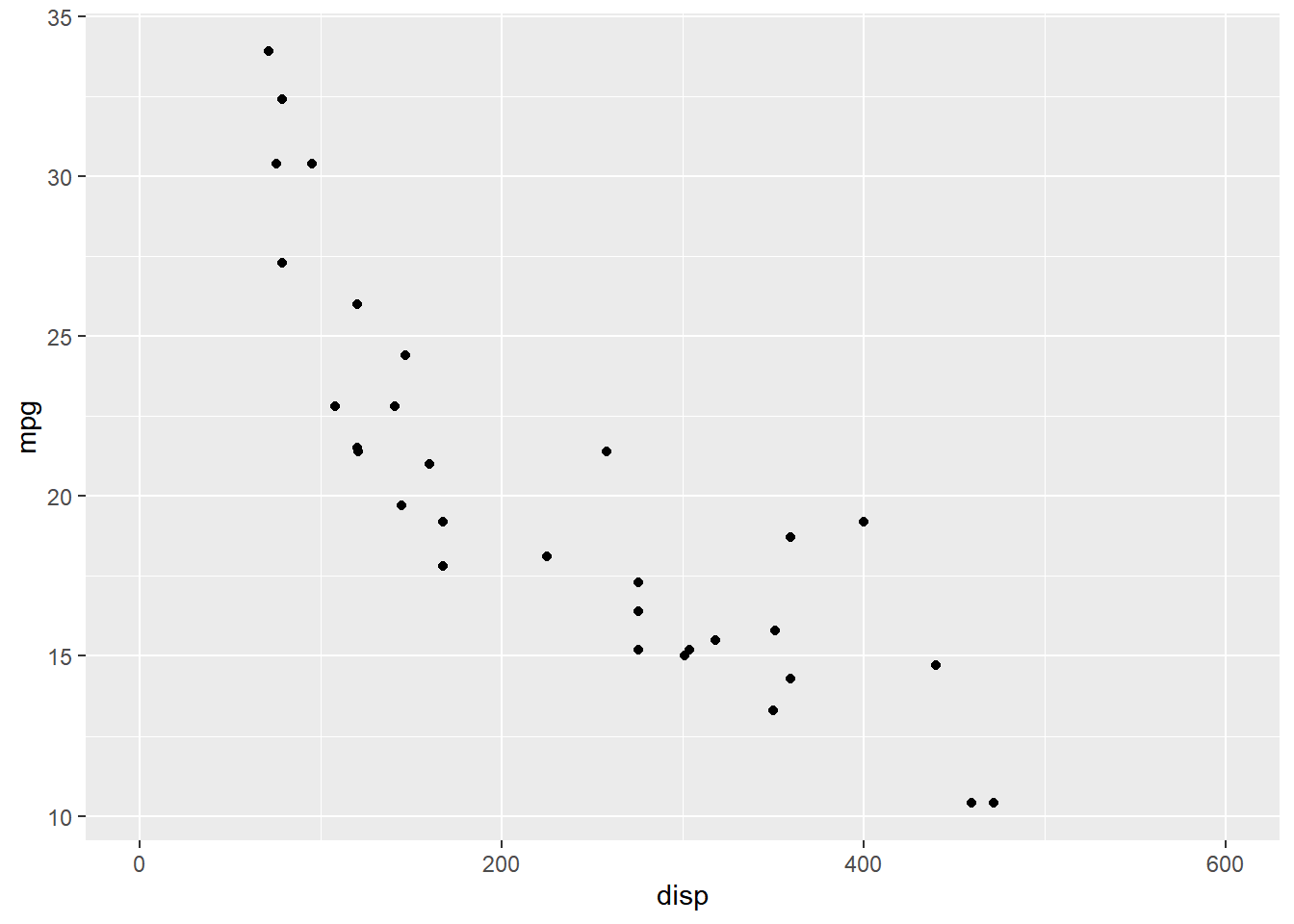### 4.6.2 Y Axis

Let us limit the range of the Y axis between `0` and `40`.

``````ggplot(mtcars) +
geom_point(aes(disp, mpg)) +
ylim(c(0, 40))``````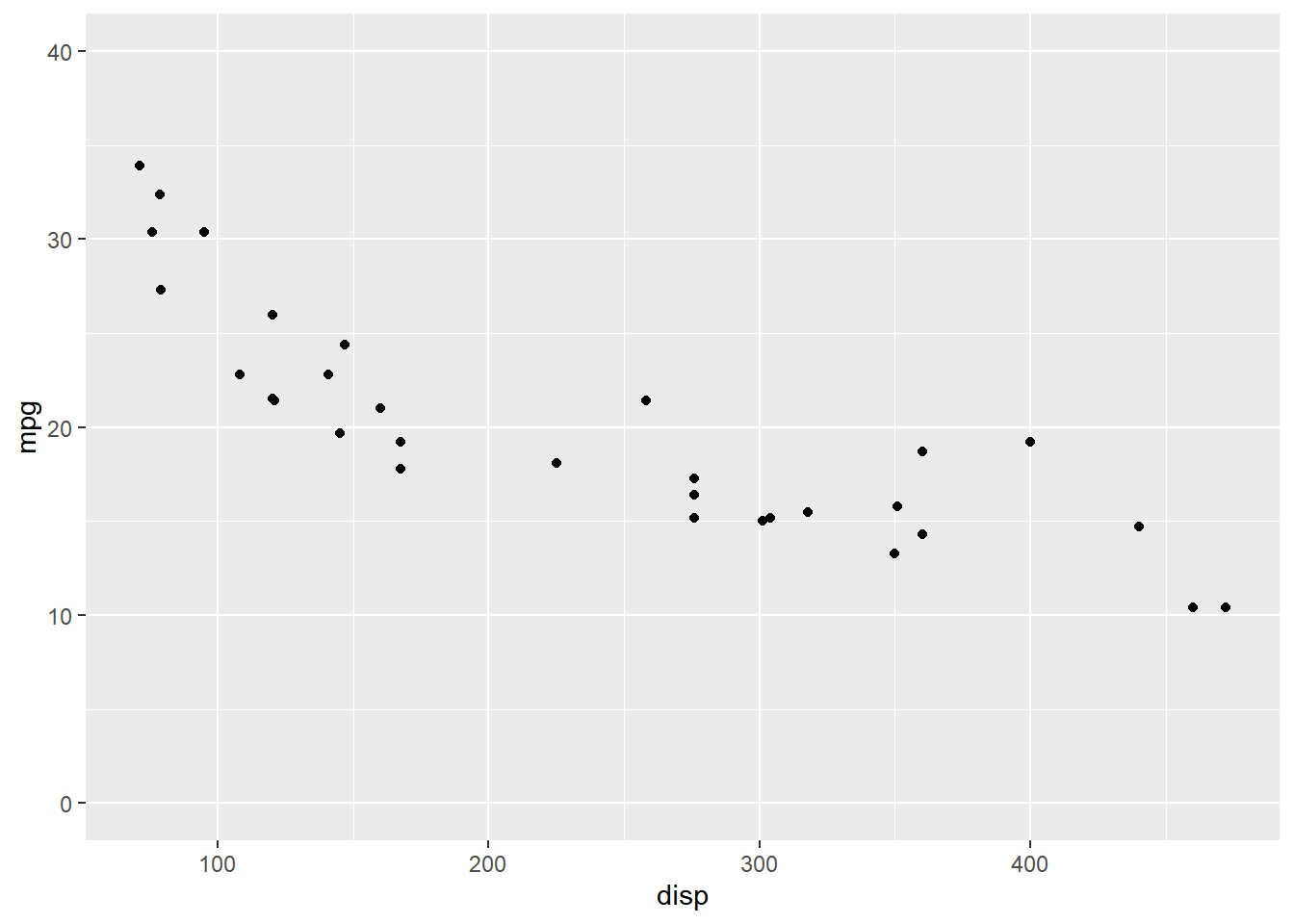### 4.6.3 Expand Limits

Let us use `expand_limits()` to limit the range of both the X and Y axis. The first input is the range for the X axis and the second input for the Y axis. In both the cases, we use a numeric vector of length 2 to specify the lower and upper limit.

``````ggplot(mtcars) +
geom_point(aes(disp, mpg)) +
expand_limits(x = c(0, 600), y = c(0, 40))``````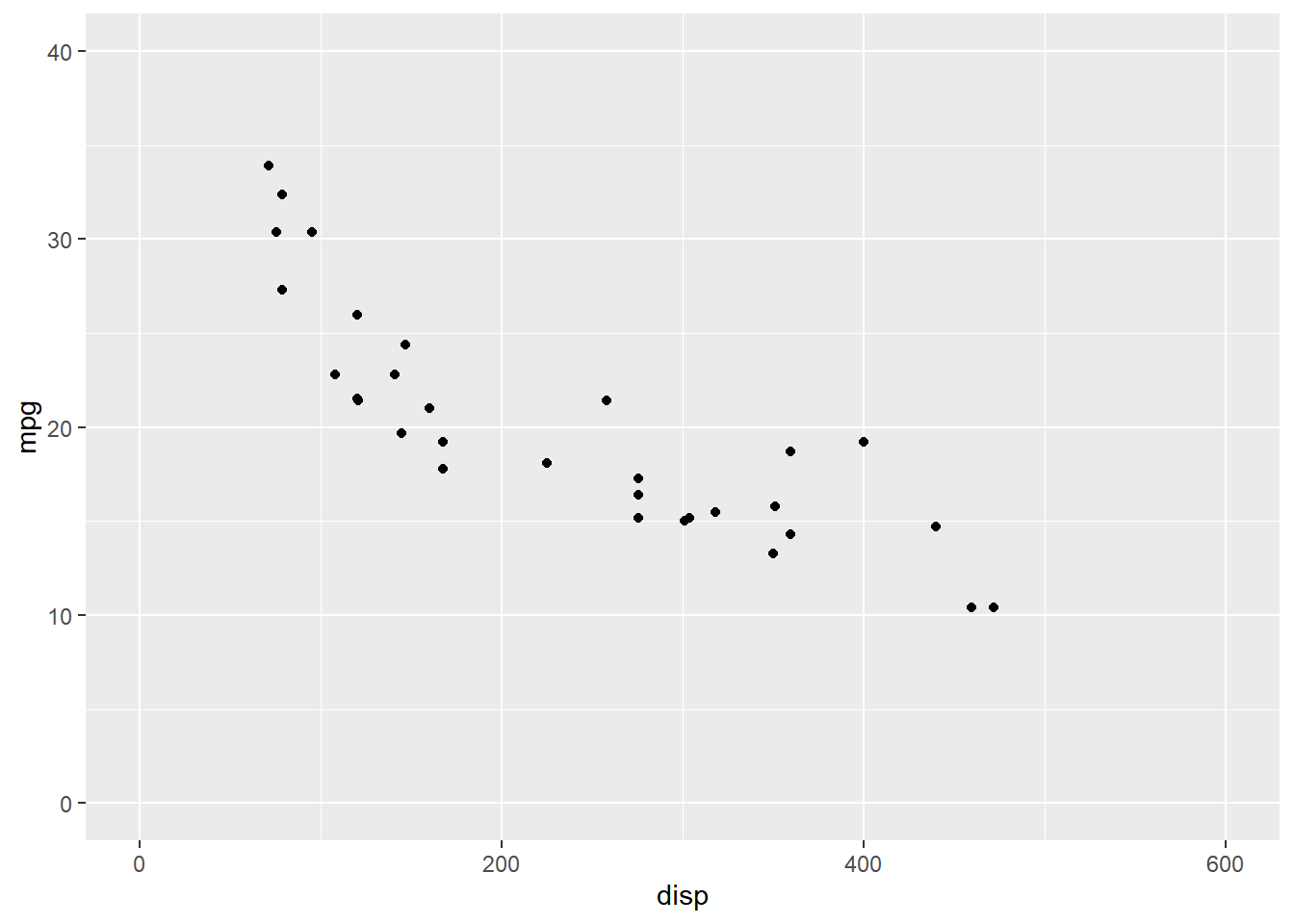### 4.6.4 Remove Axis Labels

If you want to remove the axis labels all together, use the `theme()` function. It allows us to modify every aspect of the theme of the plot. Within `theme()`, set the following to `element_blank()`.

• `axis.title.x`
• `axis.title.y`

`element_blank()` will remove the title of the X and Y axis.

``````ggplot(mtcars) +
geom_point(aes(disp, mpg)) +
theme(axis.title.x = element_blank(), axis.title.y = element_blank())``````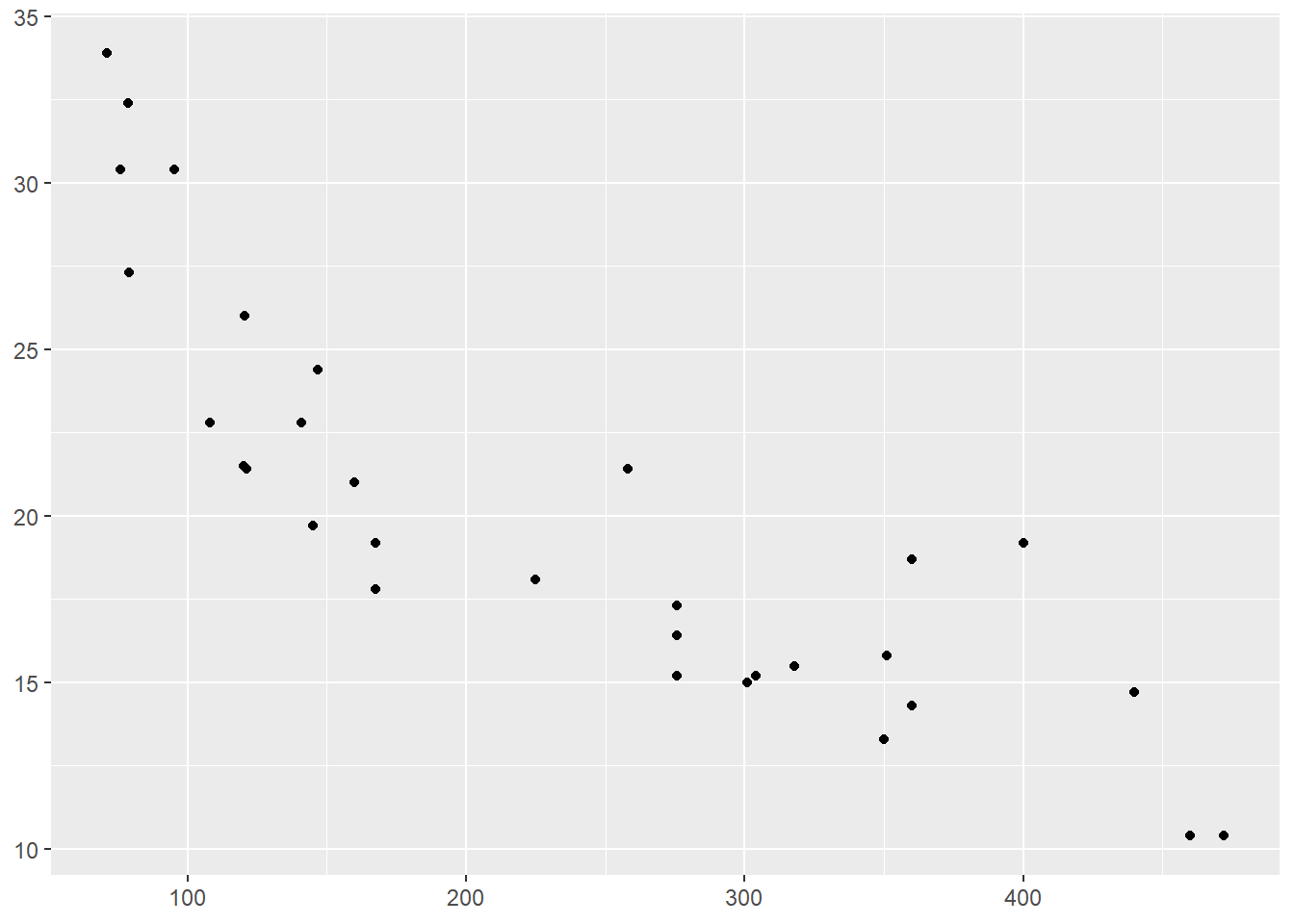## 4.7 Format Title & Axis Labels

To format the title or the axis labels, we have to modify the theme of the plot using the `theme()` function. We can modify:

• color
• font family
• font face
• font size
• horizontal alignment
• and angle

In addition to `theme()`, we will also use `element_text()`. It should be used whenever you want to modify the appearance of any text element of your plot.

### 4.7.1 Color

In the below example, we use the `color` argument within `element_text()` to modify the color of the title of the plot to `'blue'`.

``````ggplot(mtcars) +
geom_point(aes(disp, mpg)) + ggtitle('Diaplacement vs Mileage') +
theme(plot.title = element_text(color = 'blue'))``````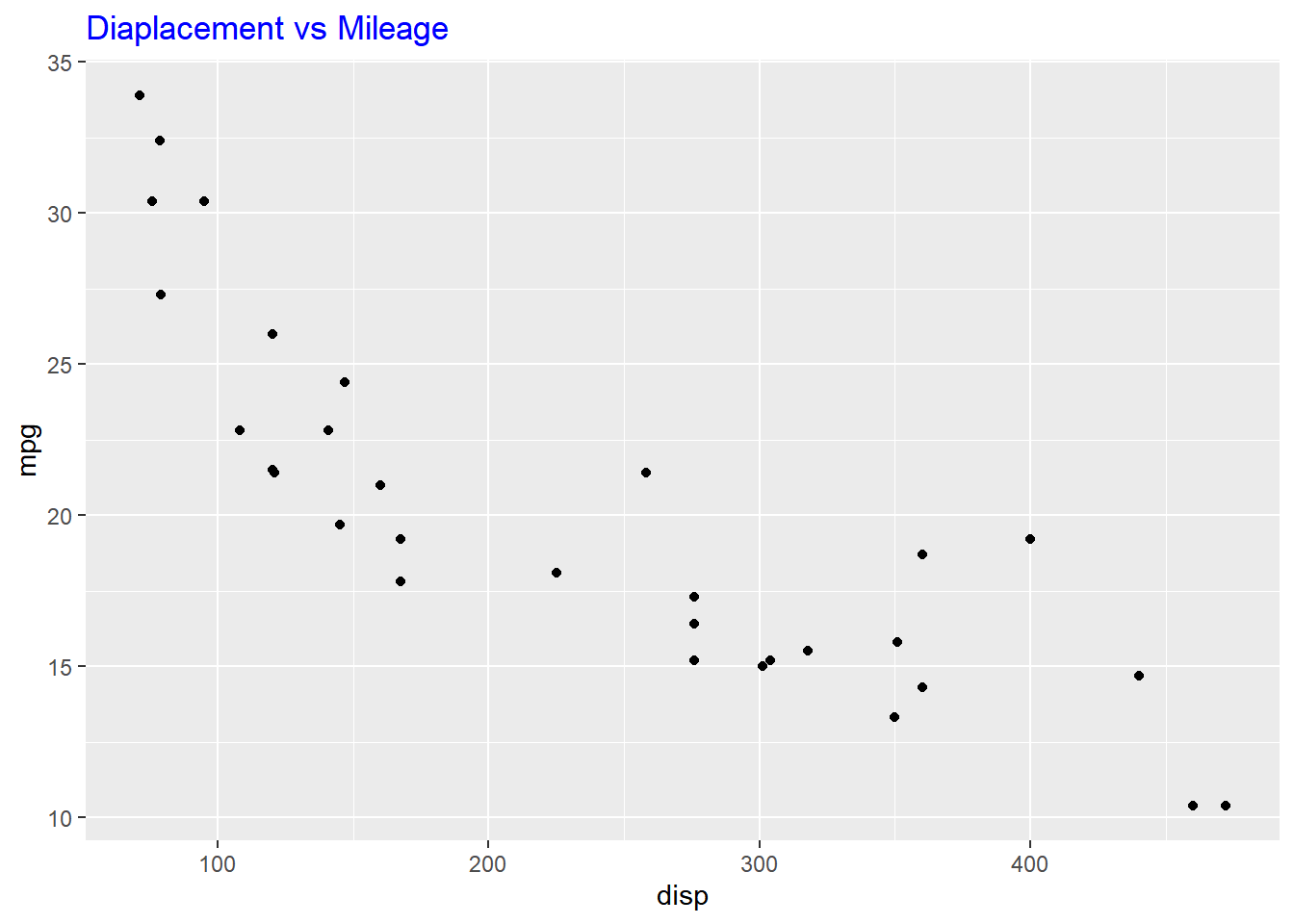### 4.7.2 Font Family

Let us change the font family of the plot title to `'Arial'` by using the `family` argument.

``````ggplot(mtcars) +
geom_point(aes(disp, mpg)) + ggtitle('Diaplacement vs Mileage') +
theme(plot.title = element_text(family = 'Arial'))``````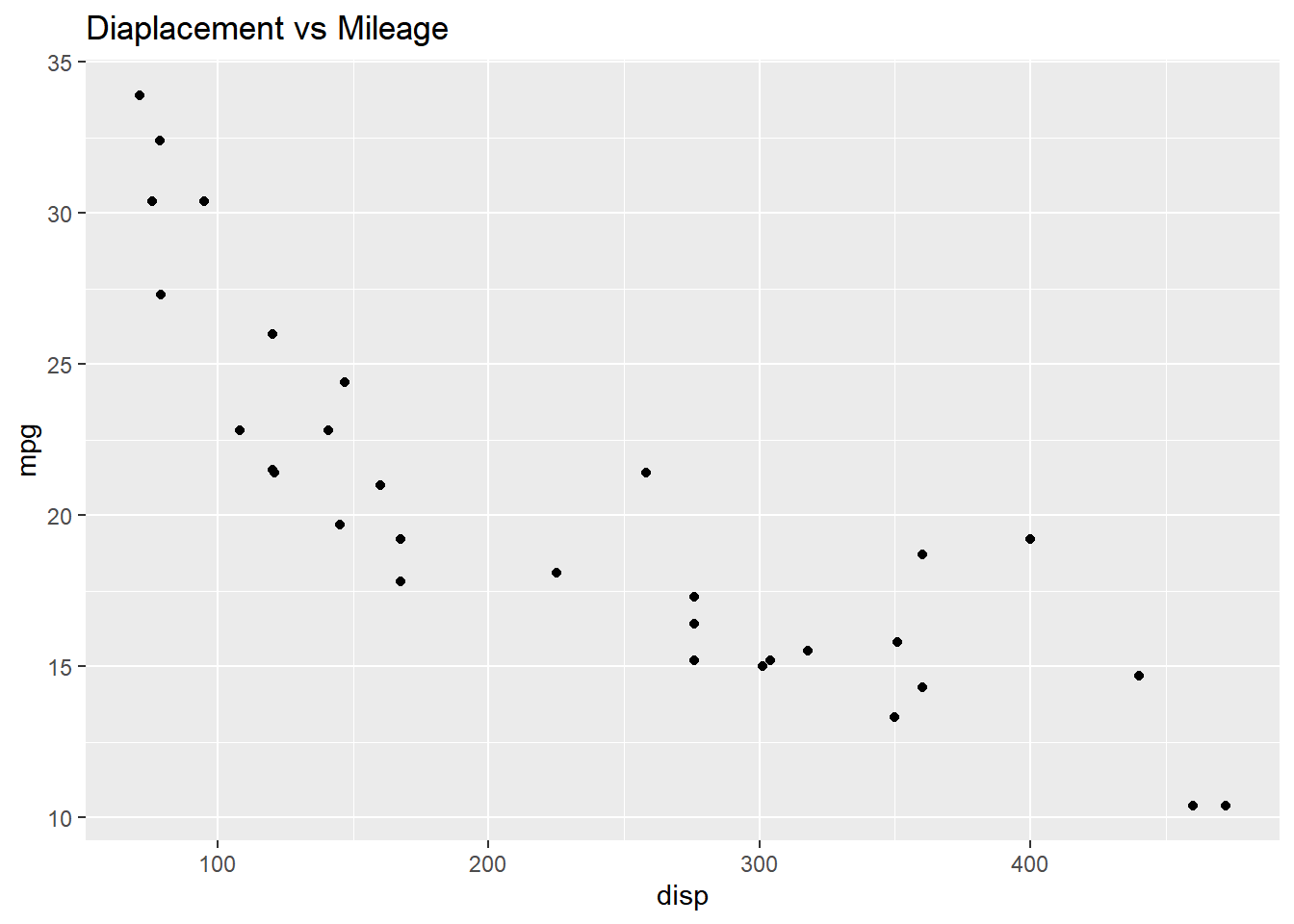### 4.7.3 Font Face

The font face can be any of the following:

• `plain`
• `bold`
• `italic`
• `bold.italic`

The `face` argument can be used to modify the font face of the title of the plot.

``````ggplot(mtcars) +
geom_point(aes(disp, mpg)) + ggtitle('Diaplacement vs Mileage') +
theme(plot.title = element_text(face = 'bold'))``````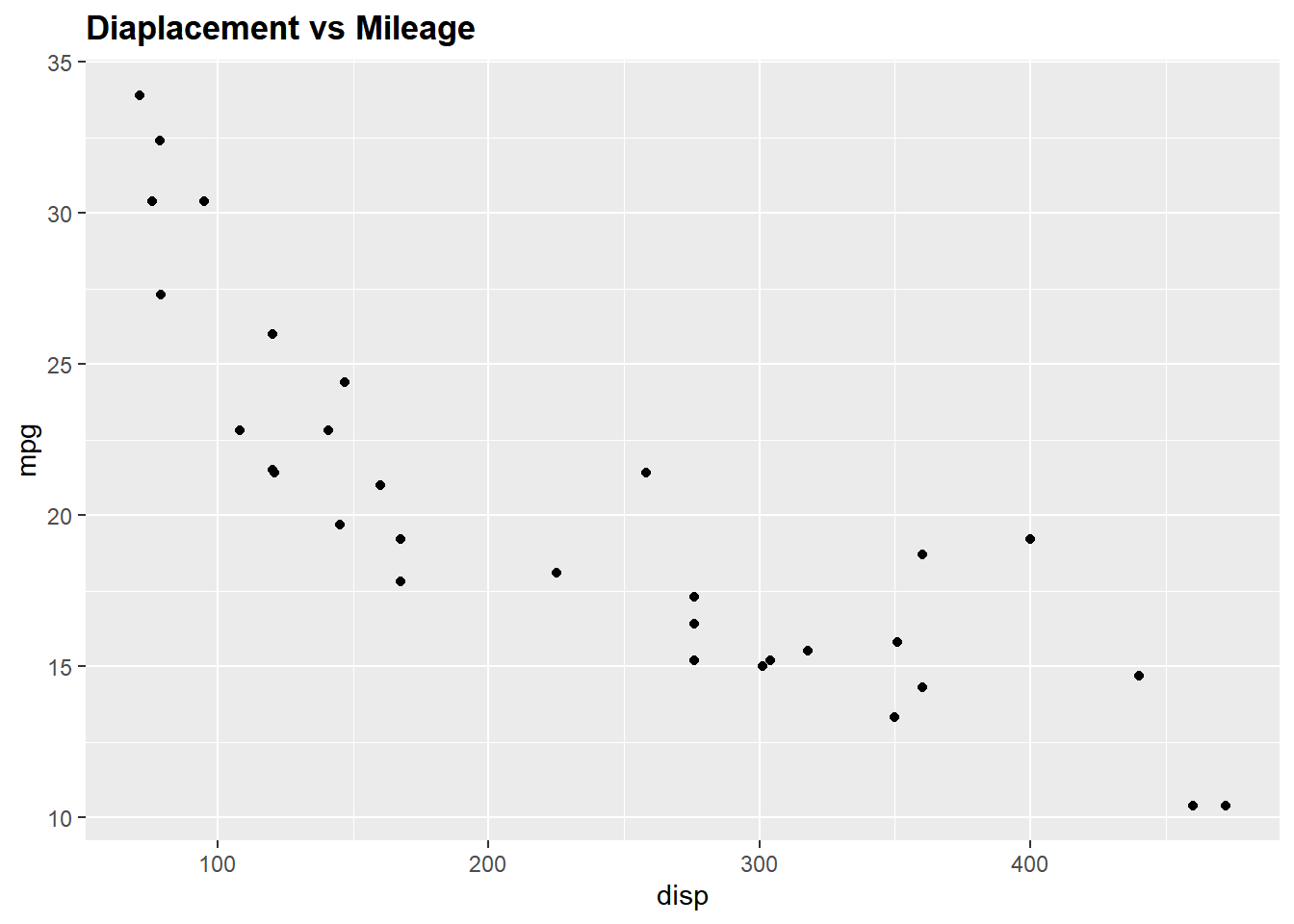### 4.7.4 Font Size

The size of the title of the plot can be modified using the `size` argument.

``````ggplot(mtcars) +
geom_point(aes(disp, mpg)) + ggtitle('Diaplacement vs Mileage') +
theme(plot.title = element_text(size = 8))``````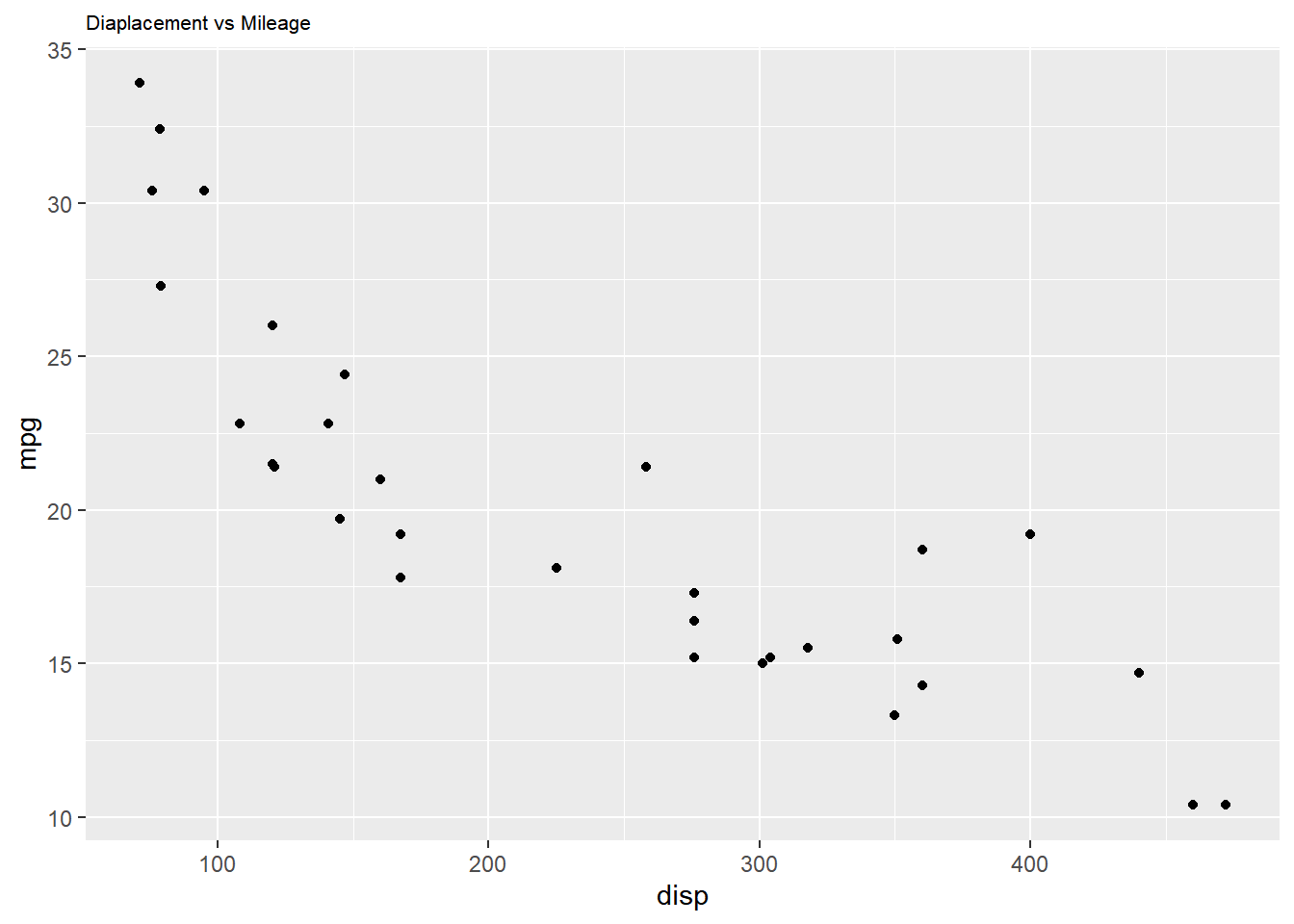## 4.8 Horizontal Alignment

To modify the horizontal alignment of the title, use the `hjust` argument. It can take values between `0` and `1`. If the value is closer to `0`, the text will be left-aligned and viceversa.

``````ggplot(mtcars) +
geom_point(aes(disp, mpg)) + ggtitle('Diaplacement vs Mileage') +
theme(plot.title = element_text(hjust = 0.3))``````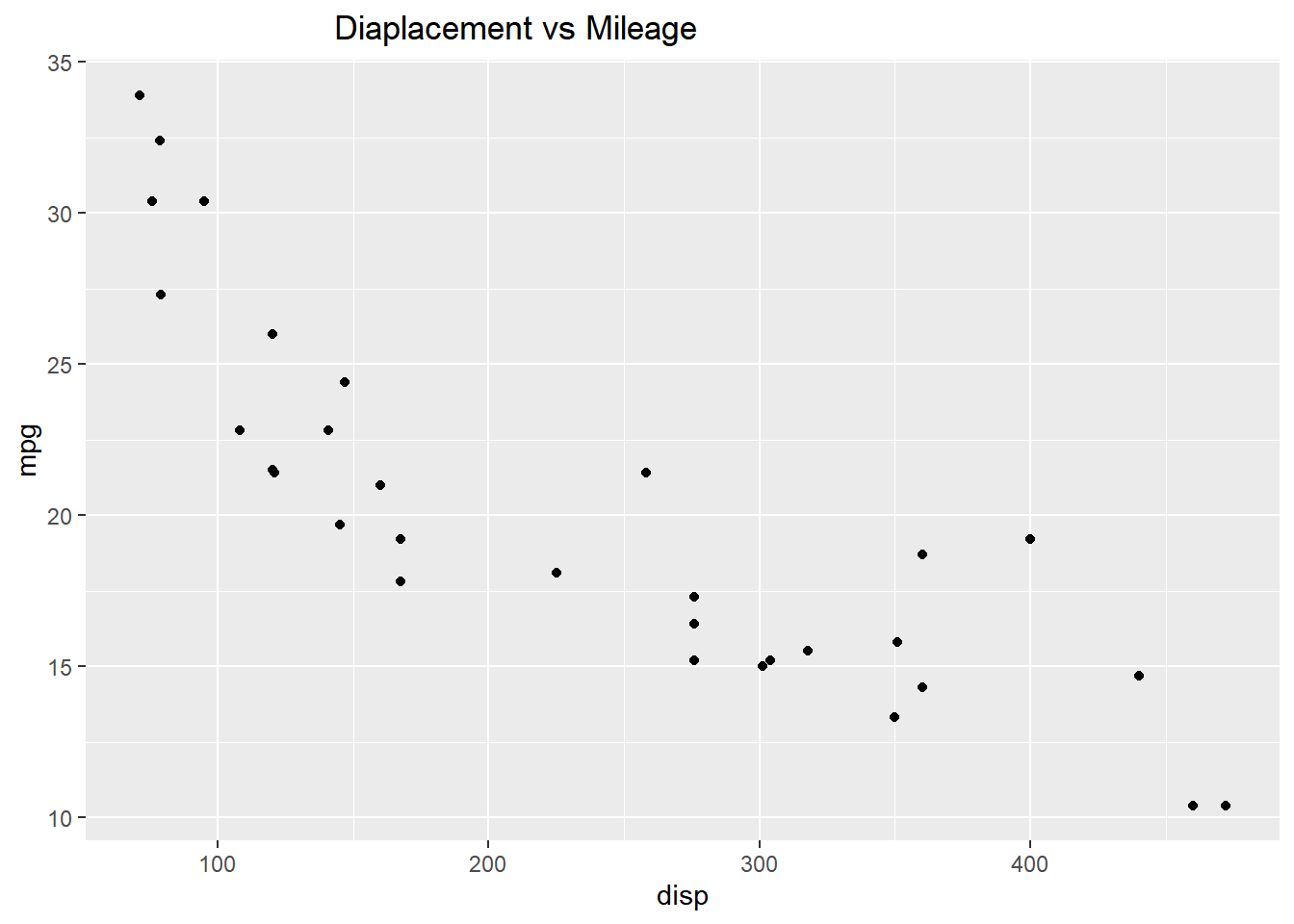## 4.9 Putting it all together…

### 4.9.1 Title

``````ggplot(mtcars) +
geom_point(aes(disp, mpg)) + ggtitle('Diaplacement vs Mileage') +
theme(plot.title = element_text(color = 'blue', family = 'Arial',
face = 'bold', size = 12, hjust = 0.5))``````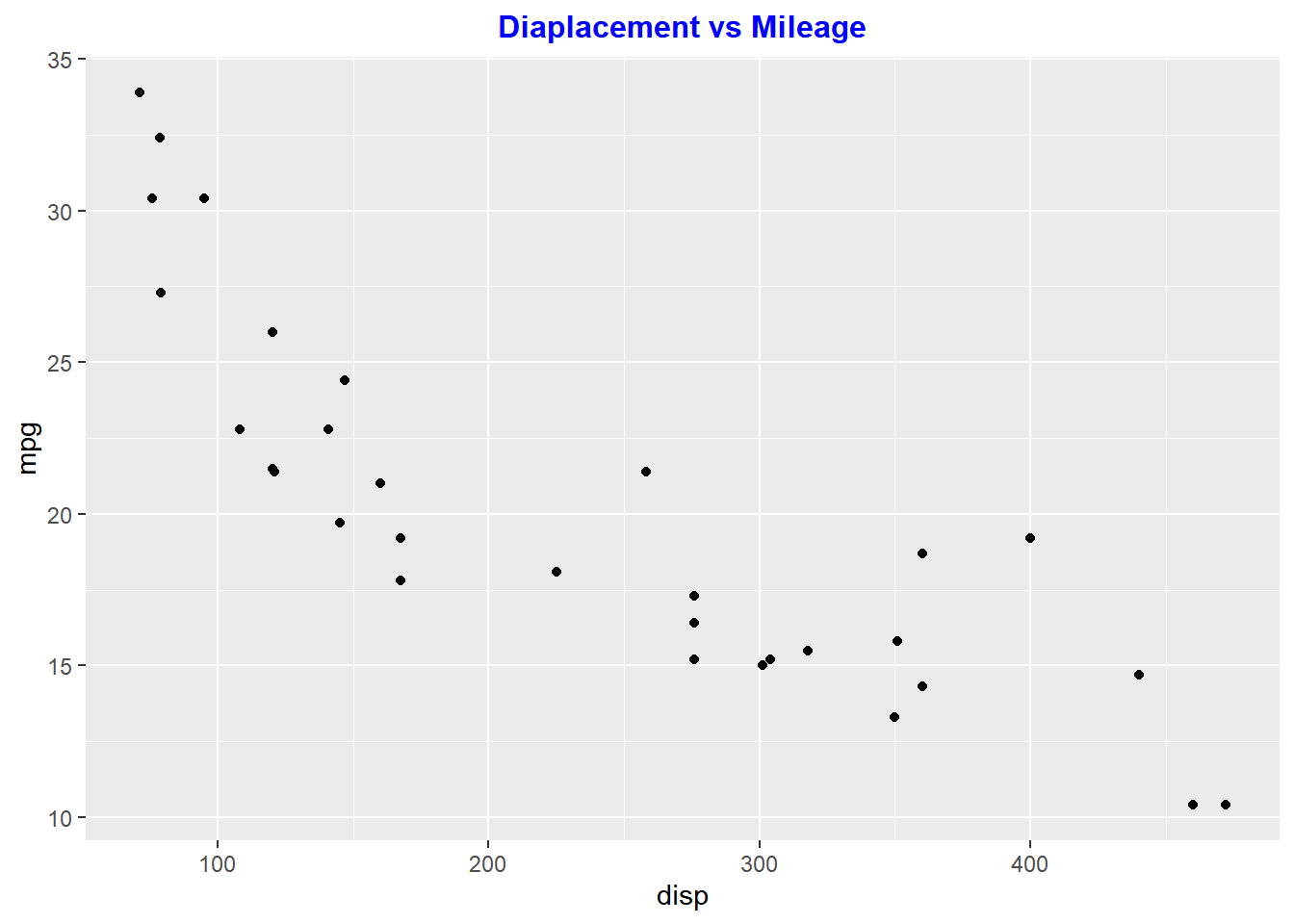### 4.9.2 X axis label

``````ggplot(mtcars) +
geom_point(aes(disp, mpg)) + xlab('Diaplacement') +
theme(axis.title.x = element_text(color = 'blue', family = 'Arial',
face = 'bold', size = 8, hjust = 0.5, angle = 15))``````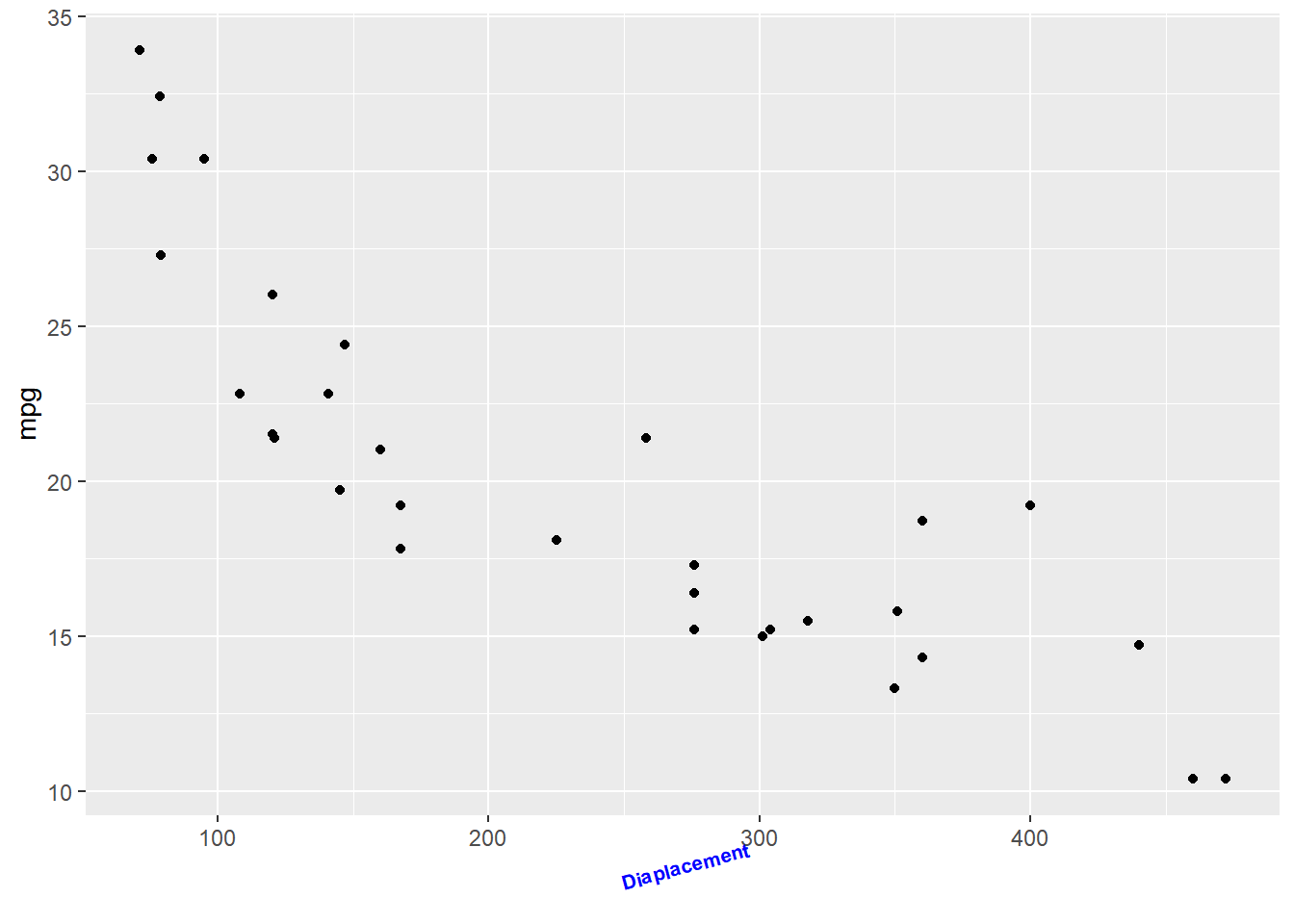### 4.9.3 Y axis label

``````ggplot(mtcars) +
geom_point(aes(disp, mpg)) + ylab('Miles Per Gallon') +
theme(axis.title.y = element_text(color = 'blue', family = 'Arial',
face = 'italic', size = 8, vjust = 0.3, angle = 90))``````